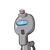# 12. The height of a particular tree is increasing at the rate of 5 cm per week. What would be the height oftree after

12. The height of a particular tree is increasing at the rate of 5 cm per week. What would be the height of
tree after 10 weeks if its present height is 10 m? [Assuming the growth of the tree is uniform.

### 1 thought on “<br />12. The height of a particular tree is increasing at the rate of 5 cm per week. What would be the height of<br />tree after”

1.Step-by-step explanation:

present height=10m

1m=100cm

so present height =1000cm

height grow in one week =5cm

height grow in 10 weeks=50cm

so total height =1000+50cm

=1050cm=10.5m